• Submissions# Portfolio Analysis in Finance Mathematics

DS Hooda*

Honorary Professor in Mathematics, GJ university of Science and Technology, India

*Corresponding author: DS Hooda, Honorary Professor in Mathematics, GJ university of Science and Technology, Hisar-125001, India

Submission: July 04, 2019;Published: November 12, 2019

DOI: 10.31031/OABB.2019.02.000546ISSN: 2578-0247
Volume2 Issue4

#### Abstract

In the present paper a brief account of the deterministic model due to Marko is given and the concept of mean variance efficient frontier to find all efficient portfolios that maximize the expected returns and minimize the variance are introduced. Some measures of portfolio analysis based on entropy meanvariance frontier and maximum entropy model in risk sharing are proposed and studied.

Keywords Risk-averse; Hyper plane; Pareto- optimal sharing; Maximum entropy principle

#### Introduction

Every investor wants to maximize his profits by selecting proper strategy for investment. There are investments like government and bank securities, real estate, mutual funds and blue chips stocks, which have low return but are relatively safe because of a proven record of non-volatility in price fluctuations. On the other hand, there are investments which bring high returns, but may be prone to a great deal of risk and the investor makes loss in case the investment goes sour [1-4].

To overcome the above mentioned problem the investor should invest his funds in a spread of low and high risk securities in such a way that the total expected return for all his investments is maximized and at the same time his risk of losing his capital is minimized. Since the various outcomes as well as the probabilities of these outcomes and the return on a unit amount invested in each security are known, therefore, there is not much difficulty in maximizing the expected return. However, the main problem is to overcome risk factor [5-8].

The earliest measure proposed regarding risk factor was variance of returns on all investments in the portfolio and was based on the argument that risk increases with variance. Markowitz  gave the concept of mean-variance efficient frontier and this enabled him to find all the efficient portfolios that maximize the expected returns and minimize the variance. Kapur & Kesavan  made a brief account of application of entropy optimization principles in minimizing risk in portfolio analysis. Hooda & Kapur  have applied these principles in characterizing crop area distributions for optimal yield.

#### Markowitz Mean-Variance-Efficient Frontier

Let j Π be the probability of jth outcome for j = 1, 2…, m and rij be the return on ith security for i = 1,2,…n, when jth outcome occurs. Then the expected return on the ith security isVariance and covariance of returns are given byA person decides to invest proportions x1, x2,…, xn of his capitals in n securities. If xi ≥ 0 for all i andthen the mean and variance of the expected returns are given by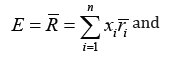Markowitz suggested that x1, x2,…, xn be chosen to maximize E and to minimize V or alternatively, to minimize V keeping E at a fixed value.wherei.e. Rj is the return on investment when jth outcome arises, and R is the mean return on investment.

Corresponding to each vector (x1, x2,…, xn), there are certain values of E and V, so that corresponding to each portfolio, there is unique point in the E-V plane. In the Figure 1 the arc AB gives the lower boundary at the convex region obtained. In this Figure 1 it can be easily seen that the portfolio corresponding to P is more efficient than the portfolio corresponding to Q because the mean return for both is the same, but variance for Q is greater than that of P. Similarly, the portfolio corresponding to P is also more efficient than the portfolio corresponding to R, because in both cases the variance is equal, while the mean return for P is higher than that for R. Thus, the portfolio corresponding to any other point on the arc AB is more efficient than a portfolio corresponding to any other point inside the convex region. However, portfolios corresponding to different points on the arc AB are not comparable, because in one portfolio the mean return may be higher, while for the other variance may be smaller. The portfolio corresponding to points of the arc AB are called mean-variance efficient frontier .

Figure 1:If a person chooses the portfolio corresponding to B it gives the highest possible value for E, but V is large at B. This means the person is interested in making his expected income large and does not mind whether variance becomes large and his risk is increased. Such persons who do not worry about risks are also known as risk prone. On the other hand, persons who want to avoid risk and are cautious are called risk-averse and they will choose points near A. Thus, the choice of point on the arc AB depends on the attitude to the risk of the investor concerned.

#### Entropy Mean-Variance Frontier

One of the investor’s objective is to diversify his portfolio so that out of all points on the mean-variance efficient frontier, he chooses that portfolio for which his investments in different stocks as equal as possible i.e. to make R1, R2,….., Rm as equal as possible among themselves. Any departure of R1, R2,….,Rm from equality is considered a measure of risk which can be minimized if we choose R1, R2,…, Rm so as to maximize the entropy measure.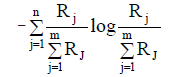Since this does not include Πj’s, therefore, we can modify the principle to say that ΠjRj’s should be as equal as possible i.e. the entropy of the probability distribution ΠjRJ should be as large as possible. For this we maximize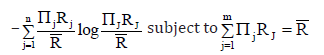Applying Lagrange’s method of multipliers, we getThus, according to our first principle Rj=R while according to second principleto be as close to the probability distribution j Π as possible. So we chose x1, x2,…, xn to minimize either D(p,Π), or D(Π,p). If we use Kullback & Leibler ’s measure, then we haveSiΣncΠe log R is constant, therefore, it implies that should be as small as possible. This is the third principle.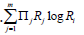Next to minimize we again apply Kullback-Leibler’s measure and getshould be as small as possible, which is fourth princissple. We can also use Harvda & Charvat ’s measure of directed divergence or cross-entropy. In that case we have to minimizeThus according to 5th and 6th principle, we choose x1, x2,…, xn to minimize respectively

Where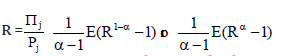#### Maximum Entropy Principle in Risk Sharing

The Pareto optimal boundary gives infinity of solutions and we need one more criterion to get a unique solution. This is possible by considering that payments are divided as uniformly as possible subject to other constraints. For this Kapur  suggested to maximize the following measure of entropy:Thus, out of all Pareto Optimal solutions we choose that one which maximizes H*.

1. Arrow KJ (1971) Essays on the theory of risk bearing. Markhan Publishers, Chicago, USA.
2. Harvda J, Charvat F (1967) Quantification method of classification processes-concept of a-entropy. Kybernetika 3(1967): 30-35.
3. Hooda DS, Kapur JN (2001) Crop area distributions for optimal yield. Opsearch 38(2001): 407-418.
4. Kapur, JN (1989) Maximum entropy models in science and engineering, Wiley Eastern Limited, New Delhi, India.
5. Kapur, JN, Kesavan HK (1992) Entropy optimization principles with applications. Academic Press INC, USA.
6. Kullback S, Leibler RA (1951) On information and sufficiency. Ann Math Stat 22(1951): 79-86.
7. Markowitz HM (1959) Portfolio selection; efficient diversification of investments. John Wiley, New York, USA.
8. Pratt JW (1964) Risk aversion in the small and in the large. Econometrics 32(1964): 122-136.
9. Raiffa H (1968) Decision analysis. Addison Wesley, USA.

﻿

We at Crimson Publishing are a group of people with a combined passion for science and research, who wants to bring to the world a unified platform where all scientific know-how is available read more...